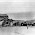# R : Converting a factor to integer

Most of R Programmers make mistake while converting a factor variable to integer.

Let's create a factor variable
a <- factor(c(2, 4, 3, 3, 4))
str(a)
Incorrect Way
a1 = as.numeric(a)
str(a1)
as. numeric() returns a vector of the levels of your factor and not the original values.

Correct Way
a2 = as.numeric(as.character(a))
str(a2)Share
Related Posts1.